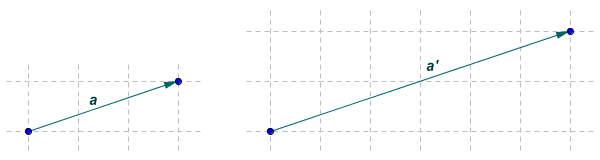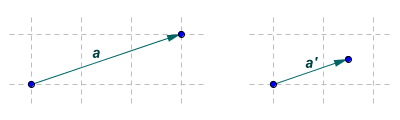# Scalar Multiplication

Multiplying a vector by a scalar (i.e. a real number) is perhaps one of the easiest operations we can perform using vectors. The process is called scalar multiplication, but is sometimes simply called scaling because, although it increases (or decreases) the magnitude of the vector, it does not alter the vector's direction. The illustration below shows a free vector a, and the new vector a that results from multiplying vector a by two.Vector a is vector a multiplied by two

Obviously, multiplying a vector by one will result in a vector that is identical to the original. Multiplying a vector by minus one, on the other hand, reverses the direction of the vector. The following illustration again shows vector a, this time multiplied by minus two.Vector a is vector a multiplied by minus two

Note that dividing a vector by a scalar is the same as multiplying it by the inverse value of the scalar. To divide a vector by two, for example, we would simply multiply it by 1/2, or 0.5. The following illustration again shows vector a, this time multiplied by 0.5.Vector a is vector a multiplied by 0.5

We can perform scalar multiplication easily using matrix arithmetic, since the operation simply requires us to multiply the x and y components (for two-dimensional vectors) or x, y and z components (for three-dimensional vectors) by the scalar. Incidentally, you may be wondering why we have chosen to use matrix arithmetic for manipulating vectors. When we manipulate vectors in some way (e.g. scaling them, moving them around, reflecting them in a line, or rotating them around some axis), we are carrying out a linear transformation. Matrices are ideally suited for representing different kinds of linear transformations in a consistent format that is easily represented as a computer data structure (for example, in the field of computer graphics). Matrix arithmetic thus allows us to combine various operations on vectors relatively easily. Here is the matrix multiplication for multiplying vector a by two:

 2a = 2 × 3 = 6 1 2